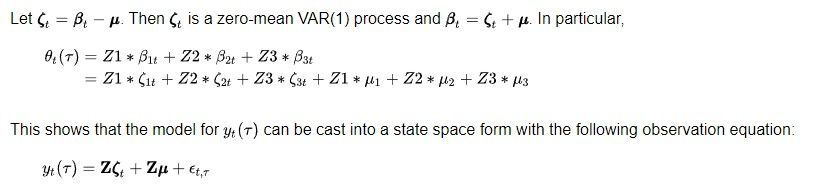## Dynamic Factor Model for the Yield Curve with exogenous variable

Hello everyone,

I'm trying to add an exogenous variable to the proc ssm in the code in example 33.7: Dynamic Factor Model for the Yield Curve.

```proc ssm data=Dns optimizer(technique=dbldog maxiter=400);
id date interval=month;

/* Time-varying parameter lambda */
parms v1-v7;
lambda = exp(v1*c1 + v2*c2 + v3*c3 + v4*c4
+ v5*c5 + v6*c6 + v7*c7);

/* Observation equation disturbance -- separate variance for each maturity */
parms sigma1-sigma17 / lower=1.e-4;
array s_array(17) sigma1-sigma17;
do i=1 to 17;
if (mtype=i) then sigma = s_array[i];
end;
if (mtype=18) then sigma = (sigma10+sigma11)/2;
irregular wn variance=sigma;

/* Variables Z1, Z2, Z3 needed in the observation equation */
Z1= 1.0;
tmp = lambda*maturity;
Z2 = (1-exp(-tmp))/tmp;
Z3 = ( 1-exp(-tmp)-tmp*exp(-tmp) )/tmp;

/* Zero-mean VAR(1) factor zeta and the associated component */
state zeta(3) type=VARMA(p(d)=1) cov(g) print=(cov ar);
comp zetaComp = (Z1-Z3)*zeta;

/* Constant mean vector mu and the associated component */
state mu(3) type=rw;
comp muComp = (Z1-Z3)*mu;

/* Observation equation */
model yield = muComp zetaComp wn;

/* Various components defined only for output purposes  */
eval yieldSurface = muComp + zetaComp;

comp zeta1 = zeta;
comp zeta2 = zeta;
comp zeta3 = zeta;
comp mu1 = mu;
comp mu2 = mu;
comp mu3 = mu;

comp z2zeta = (Z2)*zeta;
comp z3zeta = (Z3)*zeta;
comp z2Mu =  (Z2)*mu;
comp z3Mu =  (Z3)*mu;

eval beta1 = mu1 + zeta1;
eval beta2 = mu2 + zeta2;
eval beta3 = mu3 + zeta3;

eval shortTem = z2zeta + z2Mu;
eval medTerm = z3zeta + z3Mu;

/* output the component estimates and the forecasts */
output out=dnsFor pdv;
run;```

I would like to add a exogenous variable to the VARMA model, so to affect all the betas (level, slope and curvature), like in a VARMAX model.  I'm not trying only to add in the observation equation the exogenous variable, i want that the known exogenous variable affect the variable called in the example (z1-z3).

1 ACCEPTED SOLUTION

Accepted Solutions

## Re: Dynamic Factor Model for the Yield Curve with exogenous variable

If I understand your model, you could try the following:

create a zero-variable:

zero = 0;

Then modify the specification of mu and the related component as follows:

state mu(3) sinput=(refi zero zero) type=rw;

comp muComp = (Z1-Z3)*mu;

8 REPLIES 8

## Re: Dynamic Factor Model for the Yield Curve with exogenous variable

Hello,

I believe @rselukar can answer this.

Koen

## Re: Dynamic Factor Model for the Yield Curve with exogenous variable

Hello Nico98,

The VARMA type in the STATE statement of PROC SSM has relatively limited functionality. If you describe your model in more detail (all the variables in your data set, precise formulation of your SSM with these variables in mind, etc), I can try to provide the PROC SSM syntax for your model.   If you also provide some test data to along with this model, that will be even better.  Any reference article that explains/illustrates this model?

## Re: Dynamic Factor Model for the Yield Curve with exogenous variable

Hello,
thank you again for your help.
So i'm tring to model some interbanks interest rates, for example the EURIRS interest rate from 1 to 30 years. Instead of just model these yield using the Dynamic Factor Model without explanatory variable I would like to use the main refinancing rate (REFI) as exogenous variable to the VARMA part of the state space. But until now i cannot use that as explanatory variable for that specific part.
So from the example i would like to only add the effecting on an exogenous variable (REFI) on the Z1-Z2 and Z3 (so the level, slope and curvature).

## Re: Dynamic Factor Model for the Yield Curve with exogenous variable

Tell me more precisely the mathematical equations that involve your variable REFI.

## Re: Dynamic Factor Model for the Yield Curve with exogenous variable

The beta of the models are 3: beta1-beta2-beta3. With the formula in the example that is: beta1(t)=mu+zeta(t).

I want something like: beta1(t)=zu+zeta(t)+REFI(t). Where REFI is the exogenous known variable estimated outside and that give the scenario of my analysis.

## Re: Dynamic Factor Model for the Yield Curve with exogenous variable

Instead of having only the VARMA and rw process, i want also to affect the parameter (Z1-Z3) with the exogenous variable refi.From this state space you have to imagine to have also Z*REFI(t)

## Re: Dynamic Factor Model for the Yield Curve with exogenous variable

If I understand your model, you could try the following:

create a zero-variable:

zero = 0;

Then modify the specification of mu and the related component as follows:

state mu(3) sinput=(refi zero zero) type=rw;

comp muComp = (Z1-Z3)*mu;

## Re: Dynamic Factor Model for the Yield Curve with exogenous variable

Given the documentation and what i tried to do I think you are correct, so i thank you a lot.

However, the results are quite poor, so i think that just for now i will continue to use proc varmax for the betas.

Using proc ssm the path of the betas are not so much defined by the exogenous variable and are quite unreasonable.

Discussion stats
• 8 replies
• 312 views
• 1 like
• 3 in conversation